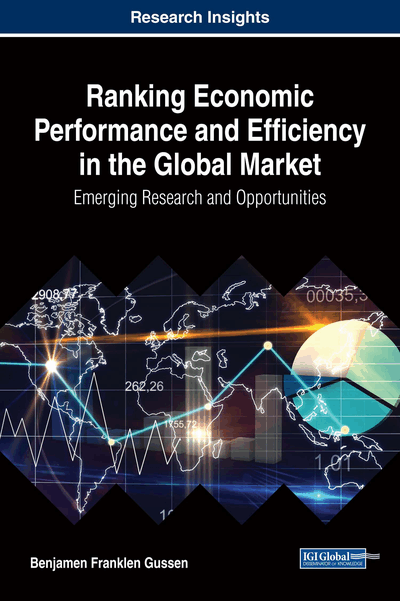# The Model

DOI: 10.4018/978-1-5225-2756-5.ch002
Available
\$37.50
No Current Special Offers

## Abstract

This chapter introduces the ? model, the analytical model used to investigate the economic efficiency of countries. The chapter provides an explanation of this model through the second law of thermodynamics, the concept of ‘complex energy,' and the principle of equipartition (from statistical mechanics). To eliminate the need for using energy units, and to enable the conversion between different input and outputs, the engineering concept of per-unit is also introduced.
Chapter Preview
Top

## Introduction

Hands being the Father, as Lands are the Mother and Womb of Wealth. (Petty, 1899, II, p. 377), cited in Georgescu-Roegen (1971, p. 280, fn 9)

As explained by Georgescu-Roegen, ‘thermodynamics is largely a physics of economic value’ (1971, p. 276). Thermodynamics, which could be traced back to Sadi Carnot (1890) and his 19th-century study of steam engines, developed from an economic problem, namely, the efficiency of steam engines. Carnot was interested in identifying the conditions under which one could obtain the highest output of mechanical work from a given input of free heat. Thermodynamics explains why the things that are useful also have an economic value. In other words, all economic value can be reduced to a common denominator: low entropy. The etymology of the word ‘entropy’ comes from the prefix ‘en’ for ‘inside,’ and the Greek root ‘tropē,’ which means ‘transformation.’ The term was coined by Rudolf Clausius (1850) to describe the amount of lost energy in an irreversible process.1 For our purposes, we define entropy as the extent of ‘spread out’ of energy in the system. Entropy is the amount of energy not available for the economic process. Low entropy suggests more free energy (available to do work), and less bounded energy. Low entropy is a necessary condition for the existence of economic value. The second law of thermodynamics, or the entropy law, states that isolated systems tend to maximize their entropy. In other words, while total energy remains constant (the first law of thermodynamics), the distribution of this energy becomes more even. The economic transformation is not automatic, as in the material domain, but depends on the actions of human beings. The economic process is distinguished by its purposive activity and its objective of ‘enjoyment of life.’ In summary, the economic process requires low entropy or free energy that is available for transforming inputs, such as land and people, to output, such as goods and services.

Georgescu-Roegen suggests that “the economic process is entropic: it neither creates nor consumes matter or energy, but only transforms low [entropy] into high entropy” (1971, p. 281). Based on this conclusion, he created a simple model of the economic process (1971, Chapters IX and X). The model includes three main inputs: land, labor, and capital. Using this model, Georgescu-Roegen explains the influence of entropy on (economic) development (1971, p. 292). It was this approach to modeling the economic process that inspired the ψ model.

The ψ model represents the economic process as a special case of energy transformation processes. The model has two inputs: land and population.2 The model uses one output, which represents all goods and services produced within a given country. The model accounts for differences between the total input energy and output energy through ‘capital,’ which is interpreted as a proxy for the efficiency of the energy transformation process over time. The energy conversion efficiency (η) is allowed to be greater than 1 through a process of accumulation from different time periods, and different space points―although this applies to microstates only. The total efficiency at the macro state, given our assumptions in relation to the ‘original condition’ (see below), is equal to 1. Capital could be interpreted as a third energy input, although it is of a different type. As detailed in the next section, capital is modeled as ‘apparent energy,’ while land and people are modeled as ‘real energy.’

Previous work on complex adaptive systems by Prigogine (1978) and others recognized that economic systems are not in ‘classical’ thermodynamic equilibrium. This realization allowed for a new conception of the entropy law. In the 1990s, the nexus between entropy and the economic process was updated through a new understanding of the law of entropy:

## Complete Chapter List

Search this Book:
Reset